### The Measurement Model for x

The second component of the LISMOD specification is the measurement model for x, as shown in the following equation: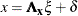The measurement model for x is similar to that for y. Assuming that x and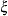are centered, this equation states that x is a function of the true scores vectorplus the error term
, which is independent of. The model matrices involved in this measurement model are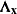(effects ofon x) and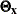, which is the covariance matrix of.

For the career aspiration data, you specify the following MATRIX statement for this measurement model:

   matrix _lambdax_ [1,1] = 0.837 0.894 0.949 0.949 0.894 0.837;


Figure 17.48 shows the output related to the specification of the measurement model for x.

Figure 17.48: Career Aspiration Analysis 3: Initial Measurement Model for x

Initial _LAMBDAX_ Matrix
f_rpa f_riq f_rses f_fses f_fiq f_fpa
rpa 0.8370 0 0 0 0 0
riq 0 0.8940 0 0 0 0
rses 0 0 0.9490 0 0 0
fses 0 0 0 0.9490 0 0
fiq 0 0 0 0 0.8940 0
fpa 0 0 0 0 0 0.8370

Initial _THETAX_ Matrix
rpa riq rses fses fiq fpa
rpa
 0
 0
 0
 0
 0
riq
 0
 0
 0
 0
 0
rses
 0
 0
 0
 0
 0
fses
 0
 0
 0
 0
 0
fiq
 0
 0
 0
 0
 0
fpa
 0
 0
 0
 0
 0
In Figure 17.48, the initial _LAMBDAX_ matrix is a 66 matrix. The _LAMBDAX_ matrix contains information about the relationships between the row indicator variables x (XVAR= variables) and the column factors(XI= variables). As specified in the MATRIX statement for _LAMBDAX_, the diagonal elements are filled with the fixed values provided. The [1,1] specification in the MATRIX statement for _LAMBDAX_ provides the starting element for the subsequent parameter list to fill in. In this case, the list contains six fixed values, and PROC CALIS proceeds from [1,1] to [2,2], [3,3] and so on until the entire list of parameters is consumed. This kind of notation is a shortcut of the following equivalent specification:
   matrix _lambdax_ [1,1]=0.837, [2,2]=0.894, [3,3]=0.949,